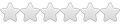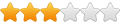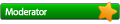## Hyperbolic COS of a complex argument

### Hyperbolic COS of a complex argument

This program computes COSH(Z) where Z is complex.
Argument entry: angle(Z) ENTER |Z| (so Y-reg = angle of Z in deg, X-reg = mag(Z))
Returns X-reg = mag(COSH(Z)) and Y-reg = angle of COSH(Z) in degrees.
The pgm changes registers 06,07,08,09, and the whole stack.

Implements Euler's definition of COSH(Z) = COSH(x + jy) = [(e^x * e^jy) + (e^-x * e^-jy)] / 2
and we say (e^x * e^jy) = a + jb, and (e^-x * e^-jy) = c + jd.

LBL COSHZ ; name of pgm
P-R ; get X=x
STO 06 ; save x
RDN
57.29578
* ; get y in degrees
STO 07
ENTER ; get Y-reg = y
RCL 06 ; get X-reg = x
e^x ; now X-reg = e^x
P-R ; now X-reg = a, Y-reg = b
STO 08 ; save a
RDN
STO 09 ; save b
RCL 07 ; get X-reg = y (still in degrees)
CHS ; we need (-y)
ENTER ; get it in Y-reg
RCL 06 ; get X-reg = x
CHS ; need (-x)
e^x ; get e^-x
P-R ; now X-reg = c, and Y-reg = d
RCL 08 ; get a
+ ; compute a+c
STO 06 ; save it
RDN ; now X-reg = d
RCL 09 ; get b
+ ; compute b+d
STO 07 ; save it. Now just get the result in polar form
RCL 06 ; now Y-reg = (b+d) and X-reg = (a+b)
R-P ; convert to polar form
2 ; don't forget to divide the mag by 2!
/
END ; end with COSH(Z) magnitude in X-reg and angle (in deg) in Y-reg.
jeff110264
.Posts: 3
Joined: Wed Oct 05, 2011 12:53 am

### Re: Hyperbolic COS of a complex argument

Hello,
since ch(x) = ( exp(x) + exp(-x) ) / 2, and the HP42 manages complex exp, what is the point ?
Why not do COMPLEX EXP ENTER 1/x + 2 / COMPLEX ?
This avoids messing with degrees and assuming RAD or DEG mode.
Can you explain ?
Regards
G.E.
gege-gege
.Posts: 1
Joined: Thu May 28, 2020 8:58 am

### Re: Hyperbolic COS of a complex argument

gege-gege wrote:Hello,
since ch(x) = ( exp(x) + exp(-x) ) / 2, and the HP42 manages complex exp, what is the point ?
Why not do COMPLEX EXP ENTER 1/x + 2 / COMPLEX ?
This avoids messing with degrees and assuming RAD or DEG mode.
Can you explain ?
Regards
G.E.

Probably because this is a site for the HP-41, which does not handle complex types, and the OP does not mention having a 42S.

Also, as that post is over 5 years old, and the OP has not visited this site since posting this, it could be some time before he/she replies.
rprosperi
......Posts: 23
Joined: Sat Apr 11, 2015 1:03 pm

### Re: Hyperbolic COS of a complex argument

because this is a site for the HP-41, which does not handle complex types

It does with Angel's 41z module!But then you'd have the ZHCOS function, written in MCode, so you don't need a user program to do it anyway.
Garth
ModeratorPosts: 210
Joined: Tue Jan 20, 2009 1:31 am